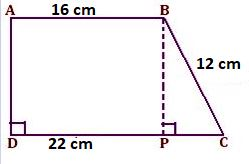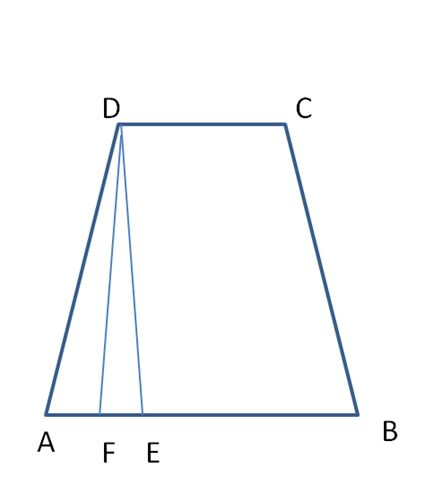# Worksheet on Trapezium | Trapezium Problems and Solutions

If you need help solving trapezium questions you can take the help of worksheets available here. Have a glance at the Worksheet on Trapezium during your practice and take your preparation to the next level. Identify the knowledge gap and allot time to the areas you are lagging. For complete guidance make use of the Trapezium Worksheets and clear all your doubts related to them.

We have given various Area of Trapezium Practice Questions and Perimeter of Trapezium Questions in the following sections. Solve all of them and check your answers to clear your doubts. For a better understanding of the concept, we have included the step by step explanation for each question.

1. The length of the parallel sides of a trapezium is in the ratio 10: 13 and the distance between them is 25 cm. If the area of trapezium is 655 cm², find the length of the parallel sides.

Solution:

Given that,

The ratio of parallel sides of the trapezium = 10: 13.

Distance between them = 25 cm

Area of trapezium = 655 cm²

Let the common ratio be x,

Then, the parallel sides are 10x, 13x

Area of trapezium formula is 1/2(a + b)h

Area of trapezium = 655 cm²

1/2(10x + 13x)25 = 655

23x . 25 = 655 . 2

575x = 1310

x = 1310/575

x = 2.278

The parallel sides of the trapezium are 10 x 2.278 = 22.78, 13 x 2.278 = 29.617

Therefore, the length of parallel sides of a trapezium are 22.78 cm, 29.617 cm.

2. Calculate the area of a trapezium whose parallel sides are 15 cm, 22 cm, and the distance between them is 26 cm.

Solution:

Given that,

The parallel sides of the trapezium are a = 15 cm, b = 22 cm

Distance between sides h = 26 cm

Area of trapezium = ½(a + b)h

A = ½(15 + 22) x 26

= 37 x 13 = 481 cm²

Therefore, the area of a trapezium is 481 cm².

3. Find the area of a trapezium whose parallel sides are 32 cm and 27 cm, and the distance between them is 15 cm.

Solution:

Given that,

The parallel sides of the trapezium are a = 32 cm, b = 27 cm

The distance between parallel sides h = 15 cm

Area of trapezium = ½(a + b)h

= ½(32 + 27)15

= ½(59) 15

= 885/2 = 442.5 cm²

Therefore, the area of a trapezium is 481 cm².

4. The area of a trapezium is 459 cm². Its parallel sides are in the ratio 7 : 10 and the perpendicular distance between them is 9 cm. Find the length of each of the parallel sides.

Solution:

Given that,

Area of a trapezium = 459 cm²

The ratio of parallel sides of the trapezium = 7 : 10

The perpendicular distance between parallel sides = 9 cm

Let the common ratio be x,

Then, the parallel sides are 7x, 10x

The area of the trapezium formula is 1/2(a + b)h

Area of trapezium = 459 cm²

1/2(a + b)h = 459

1/2(7x + 10x) 9 = 459

1/2(17x) 9 = 459

153x/2 = 459

153x = 459 x 2

153x = 918

x = 918/153

x = 6

The parallel sides of the trapezium are 7 x 6 = 42 cm, 10 x 6 = 60 cm.

Therefore, the length of each parallel side is 42 cm, 60 cm.

5. The area of a trapezium is 150 cm² and its height is 5 cm. If one of the parallel sides is longer than the other by 6 cm, find the two parallel sides.

Solution:

Given that,

The area of trapezium = 150 cm²

Height h = 5 cm

Let the parallel sides of the trapezium are a, b.

One of the parallel sides is longer than the other by 6 cm.

a = b + 6

The area of the trapezium formula is 1/2(a + b)h

Area of trapezium = 150 cm²

1/2(b + 6 + b)5 = 150

1/2(2b + 6)5 = 150

1/2(2b + 6) = 150/5

1/2(2b + 6) = 30

2b + 6 = 30 x 2

2b + 6 = 60

2b = 60 – 6

2b = 54

b = 54/2

b = 27.5 cm

a = 27.5 + 6 = 33.5 cm

Therefore, two parallel sides are 33.5 cm, 27.5 cm.

6. The area of a trapezium is 180 cm² and its height is 8 cm. If one of the parallel sides is 1.5 times the other side, find the two parallel sides.

Solution:

Given that,

Trapezium area = 180 cm²

Height h = 8 cm

Let a, b are the parallel sides of the trapezium.

One of the parallel sides is 1.5 times the other side

a = 1.5b

Area of trapezium = 1/2(a + b)h

180 = 1/2(1.5b + b)8

180 = 4(2.5b)

10b = 180

b = 180/10

b = 18

a = 1.5 x 18 = 27

Therefore, the length of parallel sides of a trapezium is 18 cm, 27 cm.

7. The area of a trapezium is 560 cm². If the lengths of its parallel sides are 25 cm and 32 cm, find the distance between them.

Solution:

Given that,

The area of trapezium = 560

Length of parallel sides a = 25 cm, b = 32 cm

Area of trapezium = 1/2(a + b)h

560 = 1/2(25 + 32)h

57h/2 = 560

57h = 560 x 2

57h = 1120

h = 1120/57

h = 19.64 cm

Therefore, the trapezium height is 19.64 cm.

8. The area of a trapezium is 1200 cm² and the distance between its parallel sides is 12 cm. If one of the parallel sides is 84 cm, find the other.

Solution:

Given that,

Trapezium area = 1200

The distance between parallel sides h = 12 cm

Parallel side a = 84 cm

Area of trapezium = 1/2(a + b)h

1200 = 1/2(84 + b)12

1200 = (84 + b)6

1200/6 = 84 + b

200 = 84 + b

b = 200 – 84

b = 116

Therefore, other parallel side of a trapezium = 116 cm.

9. The parallel sides of a trapezium are 35 cm and 20 cm. Its nonparallel sides are both equal, each being 15 cm. Find the area of the trapezium.

Solution:

ABCD is a trapezium

AB = 35 cm, CD = AE = 20 cm, BC = 15 cm and AD = 15 cm

Through C, draw CE || AD, meeting AB at E

Draw CF ⊥ AB

Now, EB = (AB – AE) = (AB – DC)

EB = 35 – 20 = 15 cm

CE = AD = 15 cm

Now, in ∆EBC, we have CE = BC = 15 cm

It is an isosceles triangle.

Also, CF ⊥ AB

So, F is the midpoint of EB.

Therefore, EF = ¹/₂ × EB = 15/2 = 7.5 cm

Thus, in right-angled ∆CFE, we have CE = 15 cm, EF = 7.5 cm

By Pythagoras’ theorem, we have

CF = [√CE² – EF²]

= √(15² – 7.5²) = √(225 – 56.25) = √(168.75)

= 12.99

Thus, the distance between the parallel sides is 12.99 cm.

Area of trapezium ABCD = 1/2 × (sum of parallel sides) × (distance between them)

= 1/2 x (35 + 20) x 12.99

= 1/2 x (55 x 12.99)

= 714.45/2 = 357.225

Hence, the area of a trapezium = 357.225 cm².

10. ABCD is a trapezium in which AB ∥ CD, AD ⊥ DC, AB = 16 cm, BC = 12 cm and DC = 22 cm. Find the area of the trapezium.Solution:

From B draw BP perpendicular DC

Therefore, AB = DP = 16 cm

So, PC = DC – DP

= (22 – 16) cm

= 6 cm

Now, area of trapezium ABCD = Area of rectangle ABPD + Area of △ BPC

△BPC is right-angled at ∠BPC

Therefore, using Pythagoras theorem,

BC² = BP² + PC²

12² = BP² + 6²

144 = BP² + 36

BP² = 144 – 36

BP² = 108

BP = √(108)

= 10.39 cm

Now, area of trapezium ABCD = Area of rectangle ABPD + Area of ∆BPC

= AB × BP + 1/2 × PC × BP

= 16 × 10.39 + 1/2 × 6 × 10.39

= 166.24 + 1/2 x 62.34

= 166.24 + 31.17

= 197.41

Therefore, area of trapezium is 197.41 cm².

11. ABCD is a trapezium in which AB is parallel to DC and angle A is equal to angle B = 40 degrees find angle C and angle D are these angles equal?

Solution:

In trapezium ABCD,

AB is parallel to DC and AD and BC are trans

∠A + ∠D = 180°

40° + ∠D = 180°

∠D = 180° – 40°

= 140°

∠C and ∠D are equal.

Yes, ∠C and ∠D are equal and its value is 140°.

12. The parallel sides of a trapezium are 25 cm and 11 cm, while its nonparallel sides are 15 cm and 13 cm. Find the area of the trapezium.

Solution:ABCD is a trapezium whose sides are

AB = 25 cm, BC = 13 cm, CD = 11 cm and DA = 15 cm

Now, draw a line DE which is parallel to BC to form a parallelogram

Hence, DE = BC

To find the area of trapezium we will add an area of triangle DAE and the area of a parallelogram

The area of △ DAE is

AE = AB – CD = 25 – 11 = 14

s = (AE + DE + DA)/2 = (14 + 13 + 15)/2

= 42/2 = 21

Area = √[s (s – a) (s – b) (s – c)]

= √[21 (21 – 14) (21 – 13) (21 – 15)]

= √[21 (7) (8) (6)]

= √(7056) = 84 cm²

Now, DF is perpendicular to AE

Area of triangle DAE = 1/2 x base x height

84 = 1/2 x 14 x h

84 = 7h

h = 84/7

h = 12

Now, area of parallelogram = 11 x 12 = 132

Area of trapezium = Area of triangle + Area of the parallelogram

= 132 + 84 = 216

Therefore, the trapezium area is 216 cm².

13. The dimensions of an isosceles trapezium are AB = 6 cm, CD = 4 cm, AD = 14 cm. Find its area.

Solution:

Given that,

AB = a = 6 cm, CD = b = 4 cm, AD = c = 14 cm

Area of an isosceles trapezium formula is 1/2 [√(c² – (a – b)²) (a + b)]

Trapezium area = 1/2 [√(c² – (a – b)²) (a + b)]

= 1/2 [√(14² – (6 – 4)²) (6 + 4)]

= 1/2 [√(196 – (-2)²) (10)]

= 1/2 [√(196 – 4 x 10)]

= 1/2(√196 – 40)

= 1/2 (√156)

= 1/2(12.48)

= 6.24 cm²

Therefore, area of an isosceles trapezium is 6.24 cm².